# HTML5 Canvas translation, scaling and rotation tutorial

## Translation

You can reposition the center (0,0) of your drawing surface by calling the translate(x,y) method. The origin of the canvas’s coordinate system is moved to the point (x,y).

## translate(x,y) Method

The translate() method is used to move the origin point to a specified point in a canvas.

Syntax :

`ctx.translate(x, y)`
Parameters Type Description
x number The value to add to horizontal (or x) coordinates.
y number The value to add to vertical (or y) coordinates.

Example: HTML5 Canvas translation

The following web document uses the translate(x,y) method and creates two similar squares at (0,0) origin and (50,50) origin.

Output :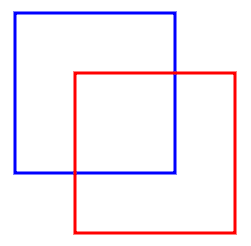Code :

``````<!DOCTYPE html>
<html>
<title>HTML5 Canvas - translate</title>
<body>
<canvas id="DemoCanvas" width="300" height="400"></canvas>
<script>
var canvas = document.getElementById("DemoCanvas");
if (canvas.getContext)
{
var ctx = canvas.getContext('2d');
ctx.beginPath();
ctx.lineWidth = "3";
ctx.strokeStyle = "blue";
ctx.strokeRect(60, 60, 160, 160);
//Move the origin from (0,0) t0 (50,50)
ctx.translate(60, 60);
ctx.strokeStyle = "red";
ctx.strokeRect(60, 60, 160, 160);
ctx.stroke();
}
</script>
</body>
</html>
``````

## Scaling

Using context’s scale(x, y) method you can expand the canvas coordinate system. Anything you draw subsequently is made bigger or smaller by the x and y values. For example, context’s scale(2,2) doubles the size of the graphics. Similarly scale(1,.5) halves the vertical (or y-axis) values that are used in context and leaves the horizontal (or x-axis) values the same.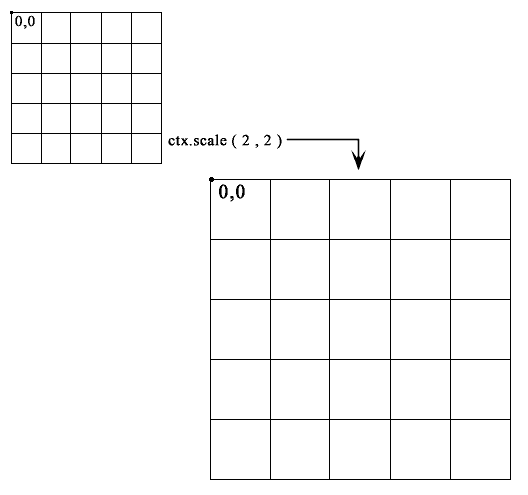## scale(x,y) Method

The translate() method is used to scale the current context by the specified horizontal (x) and vertical (y) factors.

Syntax :

```ctx.scale(x, y)
```
Parameters Type Description
x number The horizontal scaling factor, where 1 equals unity or 100% scale.
y number The vertical scaling factor.

Example : HTML5 Canvas scaling

The following web document shows scaling when it draws a rectangle.

Output :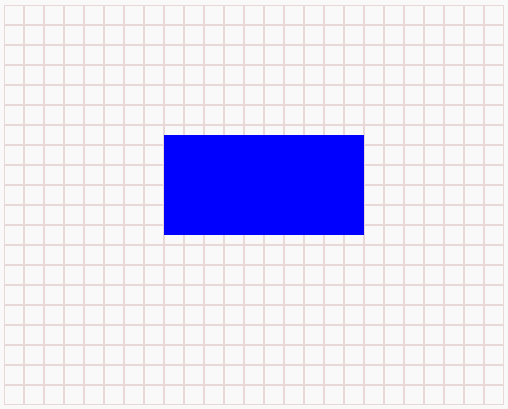Code :

``````<!DOCTYPE html>
<html>
<title>HTML5 Canvas - Scaling</title>
<body>
<canvas id="DemoCanvas" width="500" height="400"></canvas>
<script>
var canvas = document.getElementById("DemoCanvas");

if (canvas.getContext)
{
var ctx = canvas.getContext('2d');
//Modify the value of x & y and see the effect
ctx.scale(2, 2);
for (i = 0; i < 500; i += 10)
{
ctx.moveTo(0, i);
ctx.strokeStyle = "#E8D8D8";
ctx.lineTo(canvas.width, i);
ctx.stroke();

}
for (i = 0; i <511; i += 10)
{
ctx.moveTo(i, 0);
ctx.strokeStyle = "#E8D8D8";
ctx.lineTo(i,canvas.height);
ctx.stroke();
}
ctx.fillStyle = 'blue';
ctx.fillRect(80, 65, 100, 50);
}
</script>
</body>
</html>
``````

## Rotation

Using context’s rotate(angle) method you can rotate the canvas’s coordinate system around its origin. The coordinate system is rotated by angle radians, clockwise. Anything already on the canvas is unaffected, but subsequent drawing operations are rotated.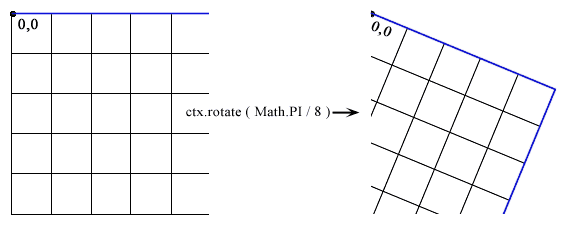## rotate(angle) Method

The rotate() method is used to rotate the current context coordinates.

Syntax :

`ctx.rotate(angle)`
Parameters Type Description
angle number The rotation angle, in radians.

Example - 1 : HTML5 Canvas rotation

The following web document draws the same rectangle before and after rotation is set.

Output: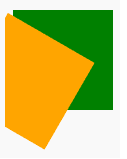Code:

``````<!DOCTYPE html>
<html>
<title>HTML5 Canvas - rotation</title>
<body>
<canvas id="DemoCanvas" width="500" height="400"></canvas>
<script>
var canvas = document.getElementById("DemoCanvas");
if (canvas.getContext)
{
var ctx = canvas.getContext('2d');

ctx.fillStyle = "green";
ctx.fillRect(8,8, 100, 100);

ctx.rotate( (Math.PI / 180) * 30);  //rotate 30    degrees.

ctx.fillStyle = "orange";
ctx.fillRect(8,8, 100, 100);
}
</script>
</body>
</html>
``````

Example - 2 : HTML5 Canvas rotation

The following web document draws two rectangles by 45-degree clockwise.

Output: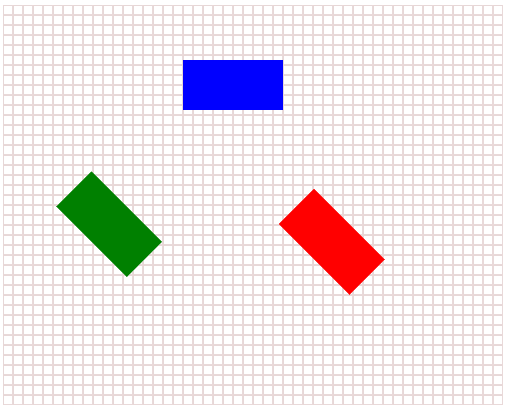Code:

``````<!DOCTYPE html>
<html>
<title>HTML5 Canvas - rotation</title>
<body>
<canvas id="DemoCanvas" width="500" height="400"></canvas>
<script>
var canvas = document.getElementById("DemoCanvas");

if (canvas.getContext)
{
var ctx = canvas.getContext('2d');
//Draw the background grid
for (i = 0; i < 500; i += 10)
{
ctx.moveTo(0, i);
ctx.strokeStyle = "#E8D8D8";
ctx.lineTo(canvas.width, i);
ctx.stroke();

}
for (i = 0; i <511; i += 10)
{
ctx.moveTo(i, 0);
ctx.strokeStyle = "#E8D8D8";
ctx.lineTo(i,canvas.height);
ctx.stroke();
}
ctx.fillStyle = 'blue';
ctx.fillRect(180, 55, 100, 50);

// rotate 45 degrees clockwise
ctx.rotate(Math.PI / 4);
ctx.fillStyle = 'green';
ctx.fillRect(180, 55, 100, 50);
ctx.fillStyle = 'red';
ctx.fillRect(350, -90, 100, 50);

}
</script>
</body>
</html>
``````

## Rotating Around a Different Point

In HTML5 canvas, all shapes rotate around the upper left corner of the canvas (0,0). Alternatively you can rotate a shape around a different point. See the following steps :

• Call context.save(). // Save the unrotated context of the canvas.
• Call context.translate(x,y). // Move to the center of the canvas to (x,y) point.
• Call context.rotate(angle). // Rotate the canvas to the specified degrees
• Draw the path, shape, or image.
• Call context.restore(). // Restore the unrotated context

In the following example, after drawing a rectangle the center of the canvas has moved (translate) to (50,10), then the canvas has rotated by 35 degrees and draws another rectangle.

Output: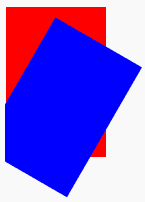Code:

``````<!DOCTYPE html>
<html>
<title>HTML5 Canvas - translate and rotation</title>
<body>
<canvas id="DemoCanvas" width="300" height="400"></canvas>
<script>
var canvas = document.getElementById("DemoCanvas");
if (canvas.getContext)
{
var ctx = canvas.getContext('2d');
// Save the unrotated context of the canvas.
ctx.save();
ctx.fillStyle = "#ff0000";
ctx.fillRect(1, 1, 100, 150);
//Move to the center of the canvas to (50,10) point.
ctx.translate(50, 10);
// Rotate the canvas by 30 degrees.
ctx.rotate( (Math.PI / 180) * 30);
ctx.fillStyle = "#0000ff";
// Draw another rectangle
ctx.fillRect(1, 1, 100, 150);
// Restore the unrotated context
ctx.restore();
}
</script>
</body>
</html>
``````

Code Editor:

See the Pen html css common editor by w3resource (@w3resource) on CodePen.